Скачать презентацию But Uncertainty is Everywhere z Medical knowledge in

747c168694732f8cd227baef94866105.ppt

• Количество слайдов: 23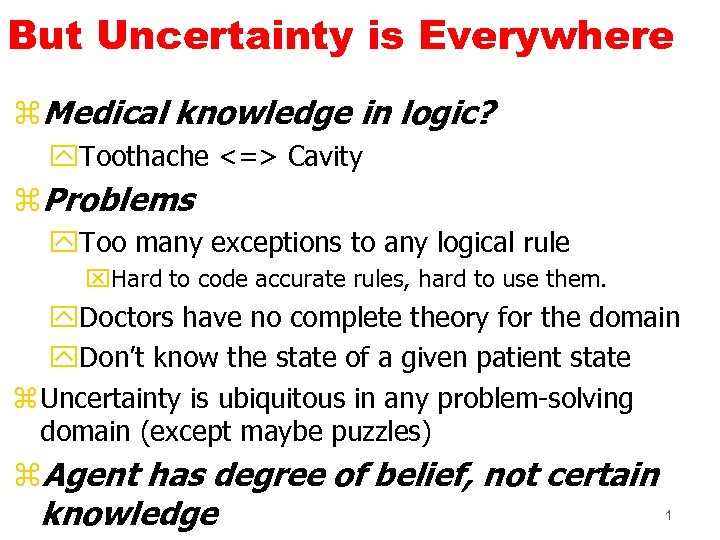But Uncertainty is Everywhere z. Medical knowledge in logic? y. Toothache <=> Cavity z. Problems y. Too many exceptions to any logical rule x. Hard to code accurate rules, hard to use them. y. Doctors have no complete theory for the domain y. Don’t know the state of a given patient state z Uncertainty is ubiquitous in any problem-solving domain (except maybe puzzles) z. Agent has degree of belief, not certain 1 knowledge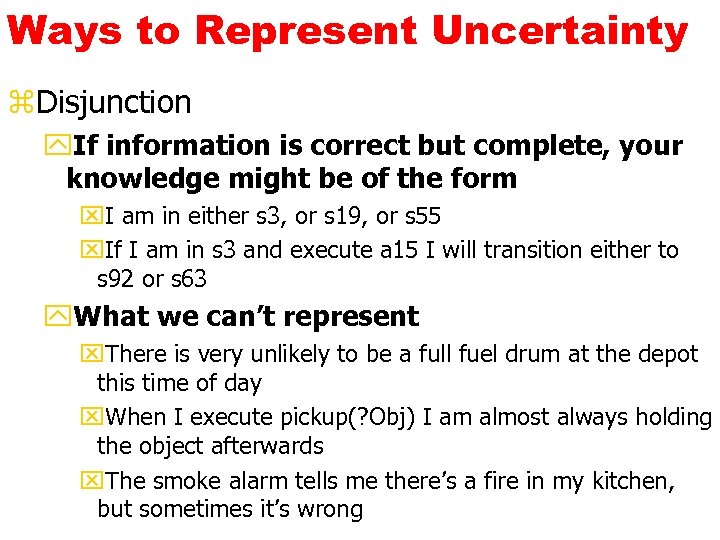Ways to Represent Uncertainty z. Disjunction y. If information is correct but complete, your knowledge might be of the form x. I am in either s 3, or s 19, or s 55 x. If I am in s 3 and execute a 15 I will transition either to s 92 or s 63 y. What we can’t represent x. There is very unlikely to be a full fuel drum at the depot this time of day x. When I execute pickup(? Obj) I am almost always holding the object afterwards x. The smoke alarm tells me there’s a fire in my kitchen, but sometimes it’s wrong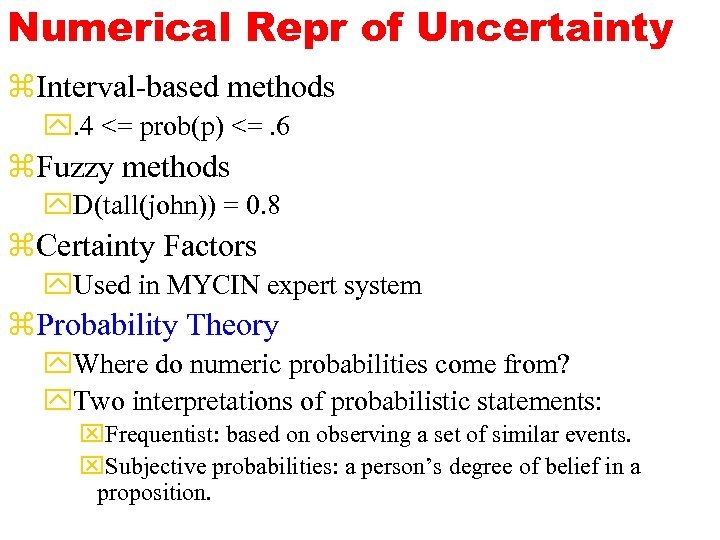Numerical Repr of Uncertainty z. Interval-based methods y. 4 <= prob(p) <=. 6 z. Fuzzy methods y. D(tall(john)) = 0. 8 z. Certainty Factors y. Used in MYCIN expert system z. Probability Theory y. Where do numeric probabilities come from? y. Two interpretations of probabilistic statements: x. Frequentist: based on observing a set of similar events. x. Subjective probabilities: a person’s degree of belief in a proposition.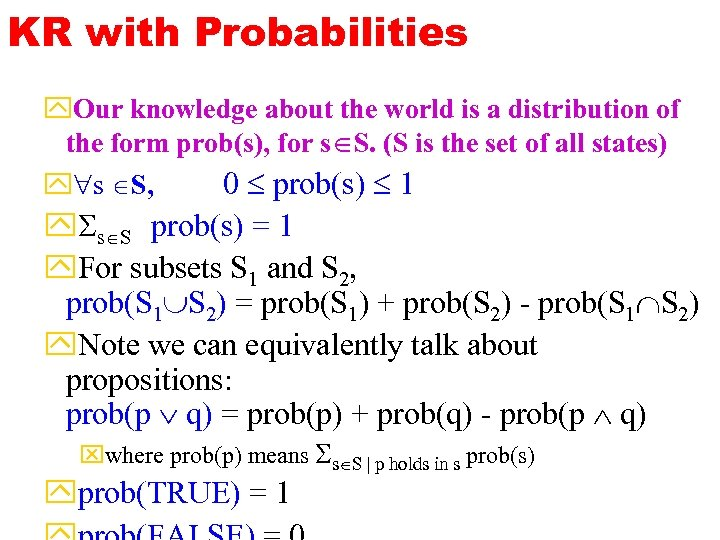KR with Probabilities y. Our knowledge about the world is a distribution of the form prob(s), for s S. (S is the set of all states) y s S, 0 prob(s) 1 y s S prob(s) = 1 y. For subsets S 1 and S 2, prob(S 1 S 2) = prob(S 1) + prob(S 2) - prob(S 1 S 2) y. Note we can equivalently talk about propositions: prob(p q) = prob(p) + prob(q) - prob(p q) xwhere prob(p) means s S | p holds in s prob(s) yprob(TRUE) = 1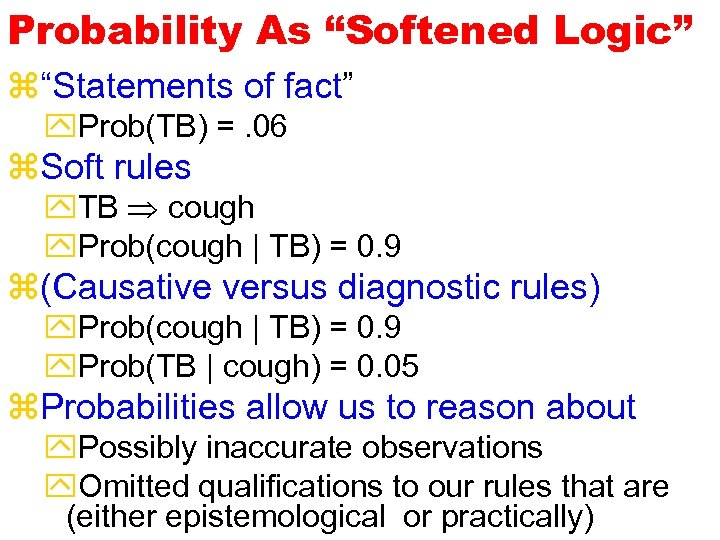Probability As “Softened Logic” z“Statements of fact” y. Prob(TB) =. 06 z. Soft rules y. TB cough y. Prob(cough | TB) = 0. 9 z(Causative versus diagnostic rules) y. Prob(cough | TB) = 0. 9 y. Prob(TB | cough) = 0. 05 z. Probabilities allow us to reason about y. Possibly inaccurate observations y. Omitted qualifications to our rules that are (either epistemological or practically)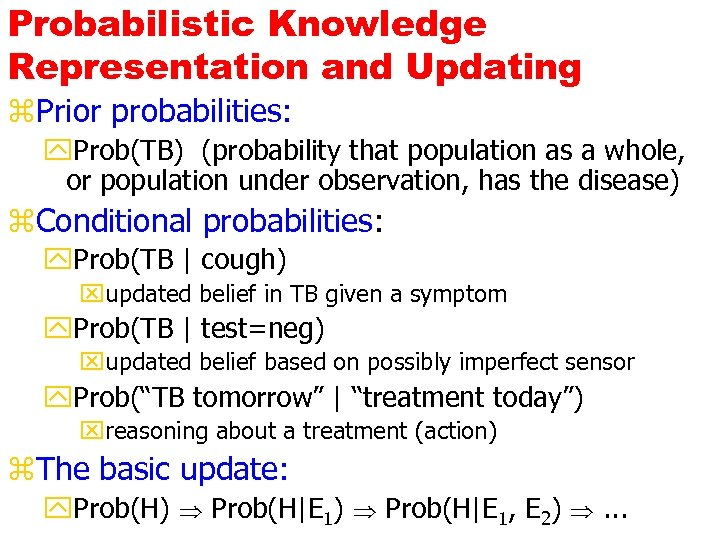Probabilistic Knowledge Representation and Updating z. Prior probabilities: y. Prob(TB) (probability that population as a whole, or population under observation, has the disease) z. Conditional probabilities: y. Prob(TB | cough) xupdated belief in TB given a symptom y. Prob(TB | test=neg) xupdated belief based on possibly imperfect sensor y. Prob(“TB tomorrow” | “treatment today”) xreasoning about a treatment (action) z. The basic update: y. Prob(H) Prob(H|E 1, E 2) . . .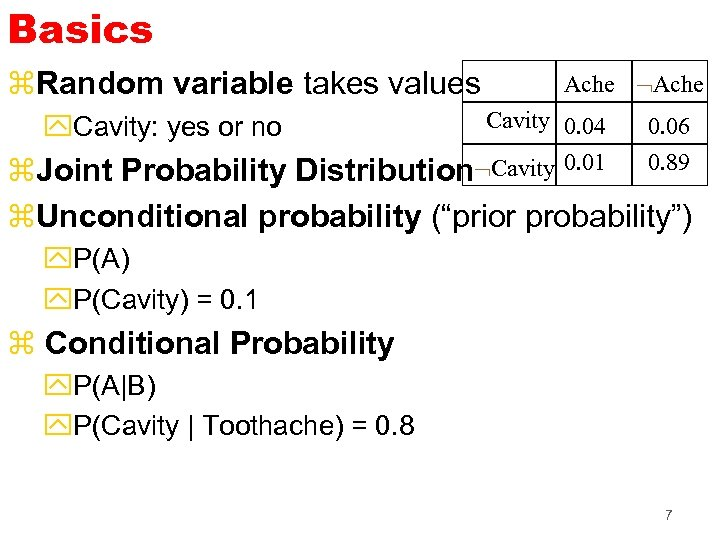Basics z. Random variable takes values y. Cavity: yes or no Ache Cavity 0. 04 Distribution Cavity 0. 01 0. 06 0. 89 z. Joint Probability z. Unconditional probability (“prior probability”) y. P(A) y. P(Cavity) = 0. 1 z Conditional Probability y. P(A|B) y. P(Cavity | Toothache) = 0. 8 7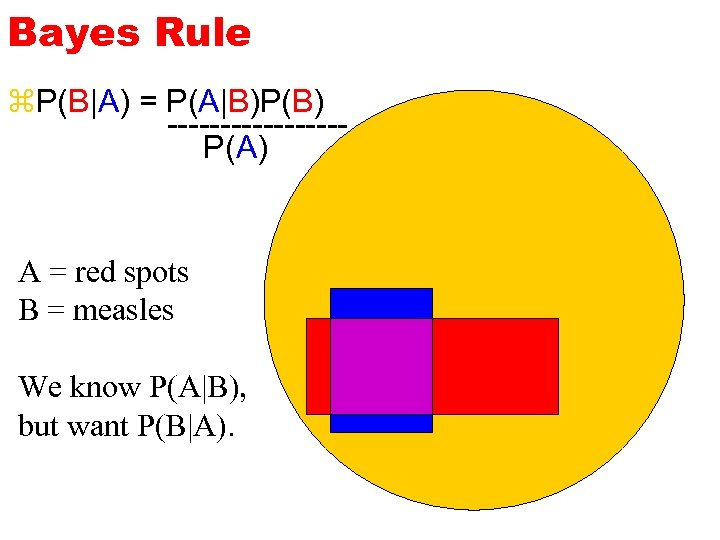Bayes Rule z. P(B|A) = P(A|B)P(B) --------P(A) A = red spots B = measles We know P(A|B), but want P(B|A).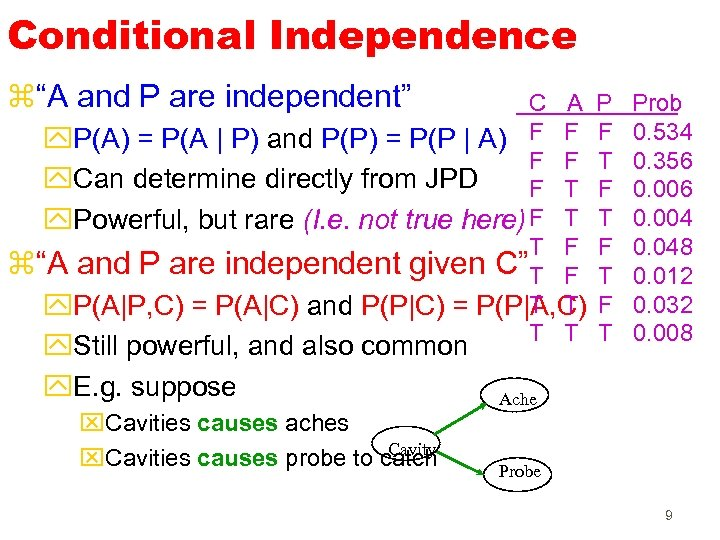Conditional Independence z“A and P are independent” C A y. P(A) = P(A | P) and P(P) = P(P | A) F F y. Can determine directly from JPD F T y. Powerful, but rare (I. e. not true here) F T T F z“A and P are independent given C”T F T T y. P(A|P, C) = P(A|C) and P(P|C) = P(P|A, C) T T y. Still powerful, and also common y. E. g. suppose x. Cavities causes aches Cavity x. Cavities causes probe to catch P F T F T Prob 0. 534 0. 356 0. 004 0. 048 0. 012 0. 032 0. 008 Ache Probe 9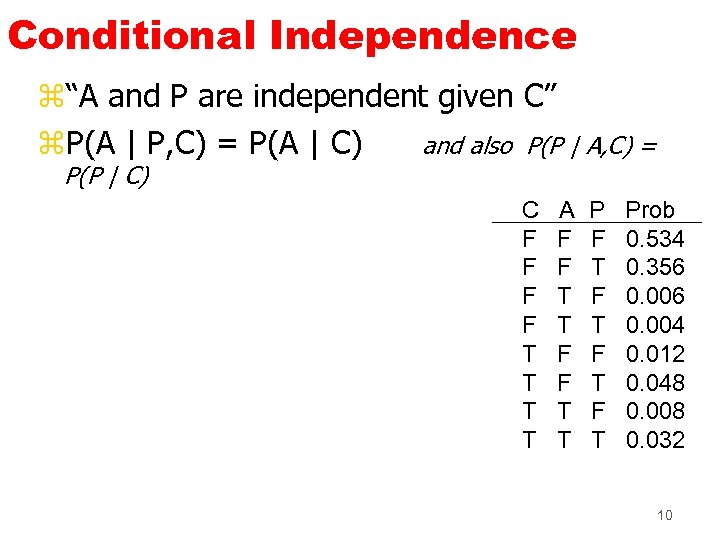Conditional Independence z“A and P are independent given C” z. P(A | P, C) = P(A | C) and also P(P | A, C) = P(P | C) C F F T T A F F T T P F T F T Prob 0. 534 0. 356 0. 004 0. 012 0. 048 0. 008 0. 032 10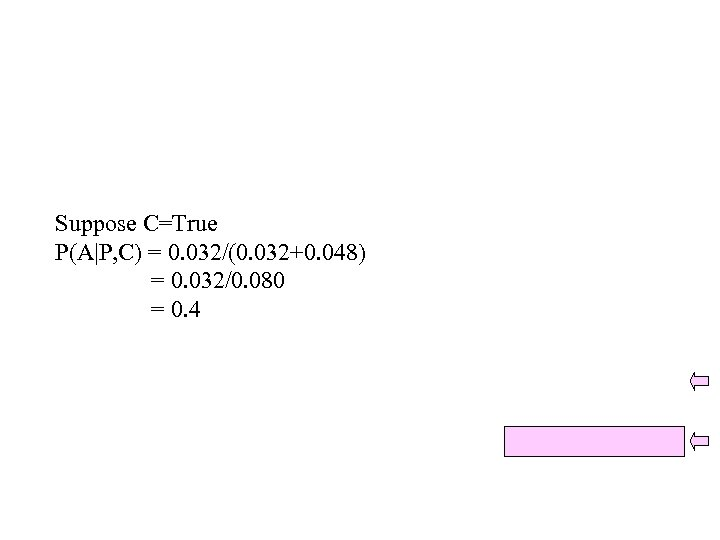Suppose C=True P(A|P, C) = 0. 032/(0. 032+0. 048) = 0. 032/0. 080 = 0. 4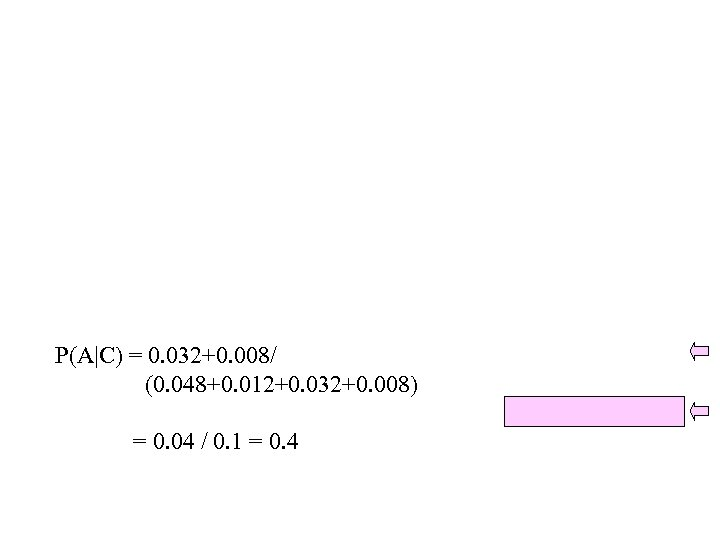P(A|C) = 0. 032+0. 008/ (0. 048+0. 012+0. 032+0. 008) = 0. 04 / 0. 1 = 0. 4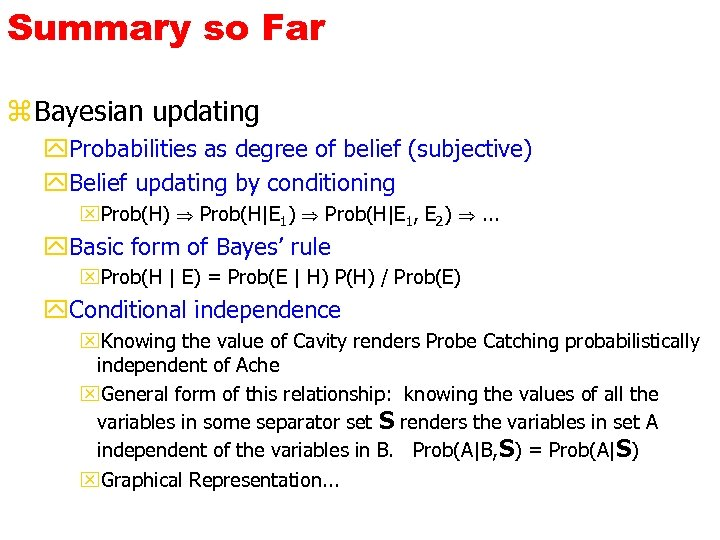Summary so Far z Bayesian updating y. Probabilities as degree of belief (subjective) y. Belief updating by conditioning x. Prob(H) Prob(H|E 1, E 2) . . . y. Basic form of Bayes’ rule x. Prob(H | E) = Prob(E | H) P(H) / Prob(E) y. Conditional independence x. Knowing the value of Cavity renders Probe Catching probabilistically independent of Ache x. General form of this relationship: knowing the values of all the variables in some separator set S renders the variables in set A independent of the variables in B. Prob(A|B, S) = Prob(A|S) x. Graphical Representation. . .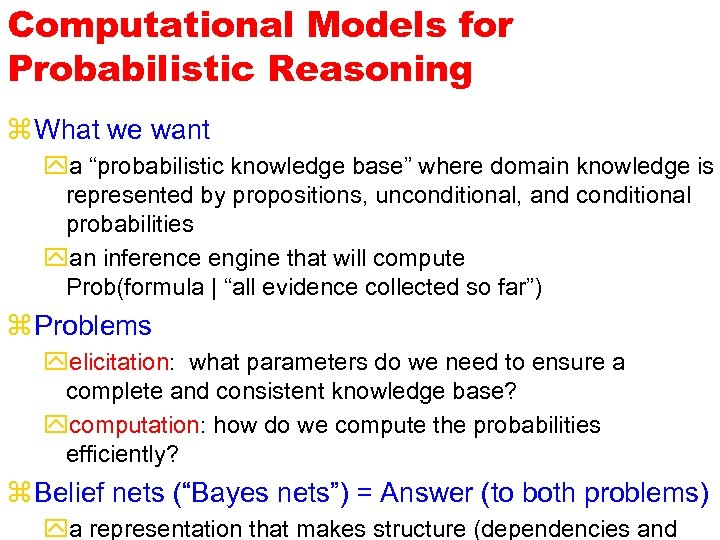Computational Models for Probabilistic Reasoning z What we want ya “probabilistic knowledge base” where domain knowledge is represented by propositions, unconditional, and conditional probabilities yan inference engine that will compute Prob(formula | “all evidence collected so far”) z Problems yelicitation: what parameters do we need to ensure a complete and consistent knowledge base? ycomputation: how do we compute the probabilities efficiently? z Belief nets (“Bayes nets”) = Answer (to both problems) ya representation that makes structure (dependencies and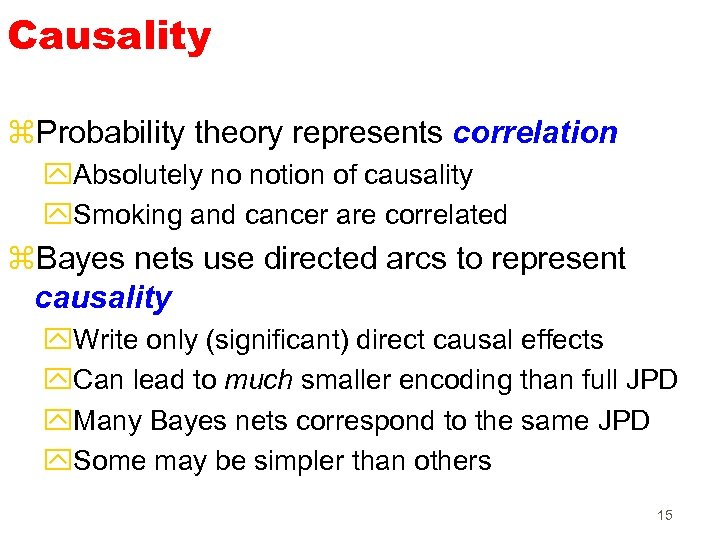Causality z. Probability theory represents correlation y. Absolutely no notion of causality y. Smoking and cancer are correlated z. Bayes nets use directed arcs to represent causality y. Write only (significant) direct causal effects y. Can lead to much smaller encoding than full JPD y. Many Bayes nets correspond to the same JPD y. Some may be simpler than others 15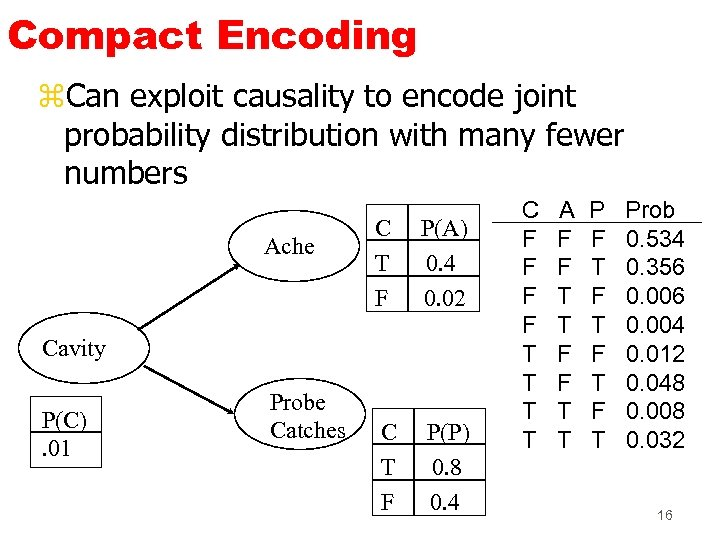Compact Encoding z. Can exploit causality to encode joint probability distribution with many fewer numbers Ache C T F P(A) 0. 4 0. 02 C T F P(P) 0. 8 0. 4 Cavity P(C). 01 Probe Catches C F F T T A F F T T P F T F T Prob 0. 534 0. 356 0. 004 0. 012 0. 048 0. 008 0. 032 16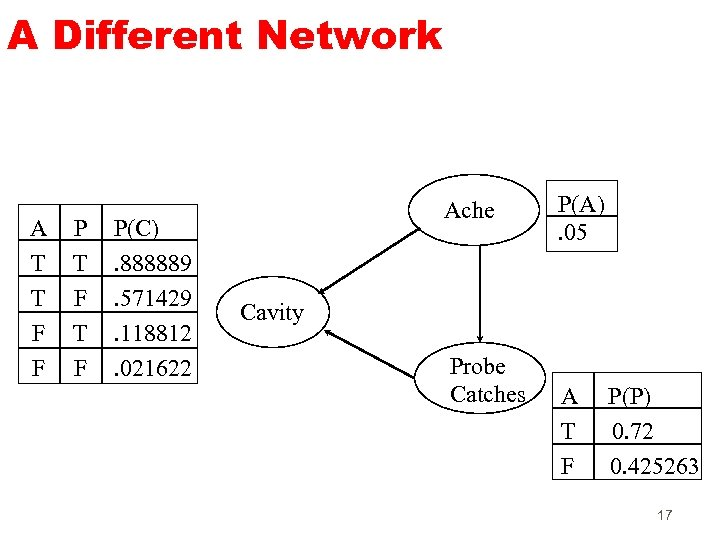A Different Network A T T F F P T F P(C). 888889. 571429. 118812. 021622 Ache P(A). 05 Cavity Probe Catches A T F P(P) 0. 72 0. 425263 17Creating a Network 1: Bayes net = representation of a JPD 2: Bayes net = set of cond. independence statements z. If create correct structure x. Ie one representing causlity y. Then get a good network x. I. e. one that’s small = easy to compute with x. One that is easy to fill in numbers 18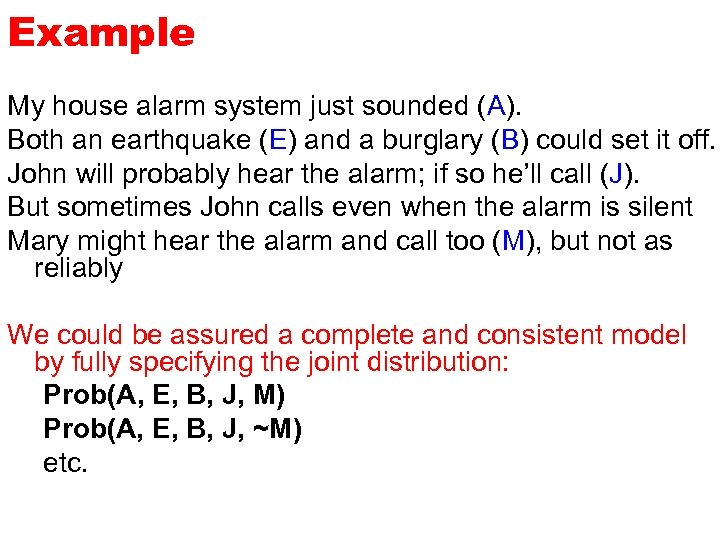Example My house alarm system just sounded (A). Both an earthquake (E) and a burglary (B) could set it off. John will probably hear the alarm; if so he’ll call (J). But sometimes John calls even when the alarm is silent Mary might hear the alarm and call too (M), but not as reliably We could be assured a complete and consistent model by fully specifying the joint distribution: Prob(A, E, B, J, M) Prob(A, E, B, J, ~M) etc.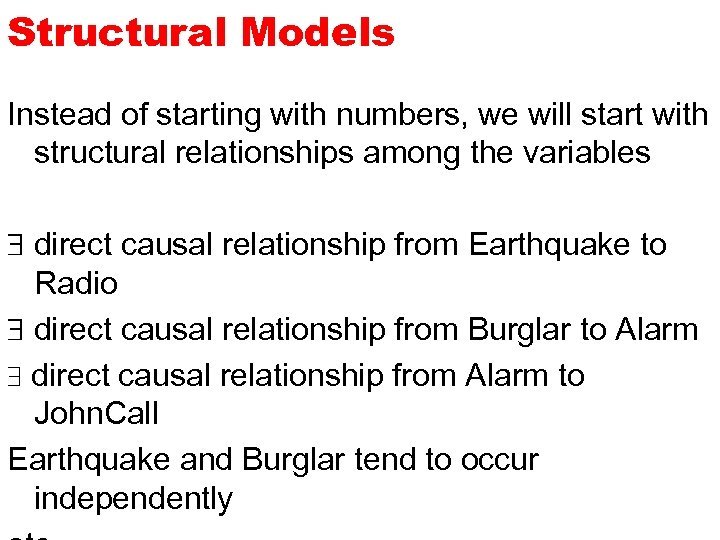Structural Models Instead of starting with numbers, we will start with structural relationships among the variables direct causal relationship from Earthquake to Radio direct causal relationship from Burglar to Alarm direct causal relationship from Alarm to John. Call Earthquake and Burglar tend to occur independently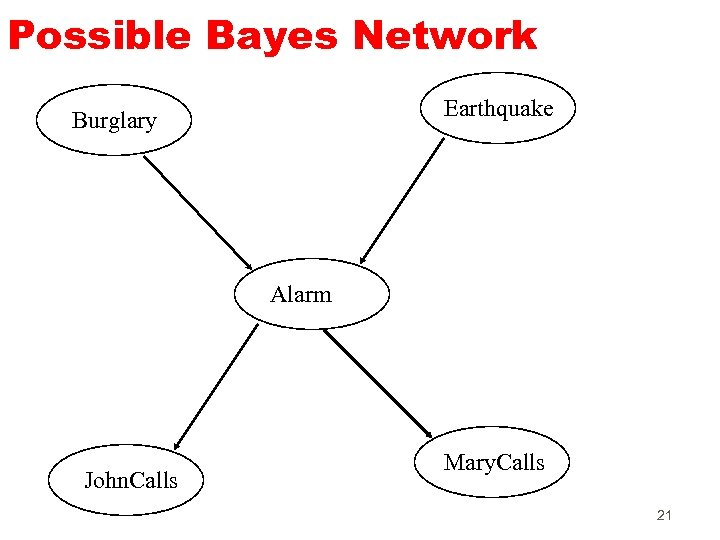Possible Bayes Network Earthquake Burglary Alarm John. Calls Mary. Calls 21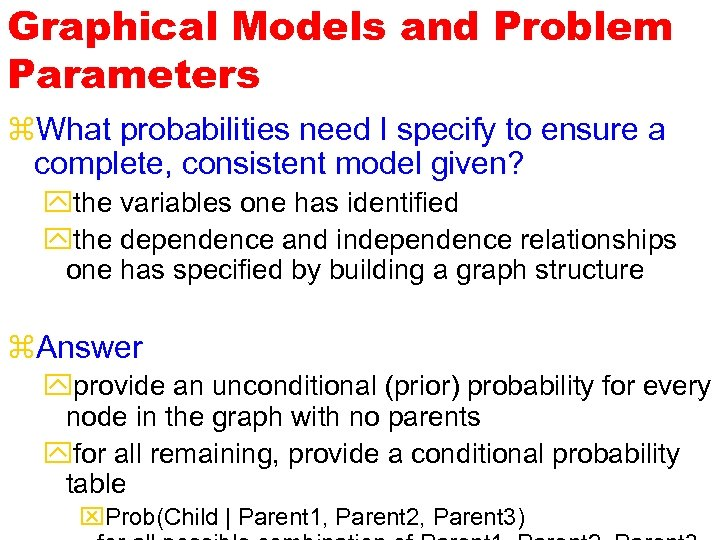Graphical Models and Problem Parameters z. What probabilities need I specify to ensure a complete, consistent model given? ythe variables one has identified ythe dependence and independence relationships one has specified by building a graph structure z. Answer yprovide an unconditional (prior) probability for every node in the graph with no parents yfor all remaining, provide a conditional probability table x. Prob(Child | Parent 1, Parent 2, Parent 3)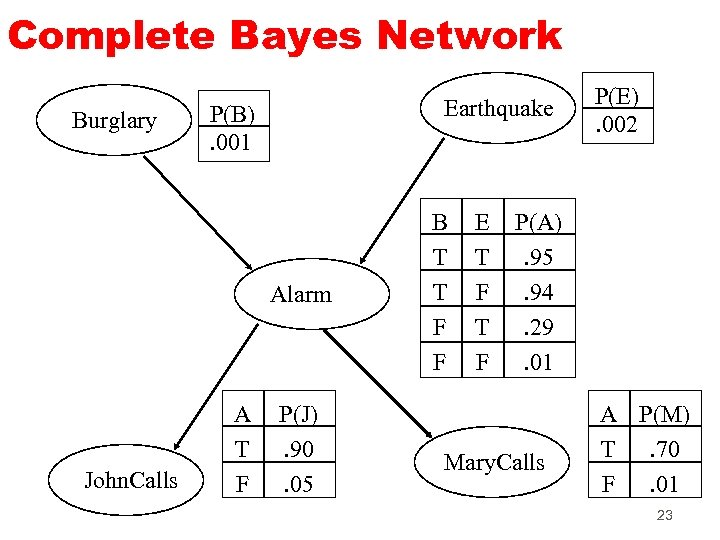Complete Bayes Network Burglary Earthquake P(B). 001 Alarm John. Calls A T F P(J). 90. 05 B T T F F E T F P(E). 002 P(A). 95. 94. 29. 01 Mary. Calls A P(M) T. 70 F. 01 23